Inequality

(a+b)(b+c)(c+a) ≥ 8abc

For any a, b and c 0 we have

(a + b)(b + c)(c + a) ≥ 8abc

Proof. We have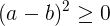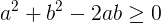We add 4ab to this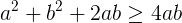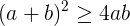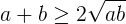And now we have the same thing here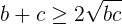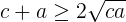a, b and c are non-negative numbers.

So, we can multiply these 3 inequalities: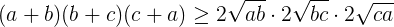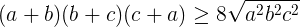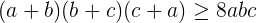Keywords: Algebraic Inequalities, algebra, inequality

Forum

Here you can discuss about mathematic, about algebra, geometry, trigonometry.

It is not mandatory to be logged in on this forum but it is nice to have an account. You can ask about mathematics just with your name and your email.

This maths forum is one of the easiest forums to use it.

>> Go to Math Forum